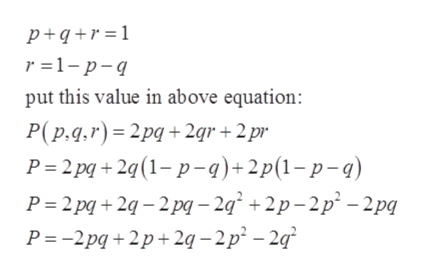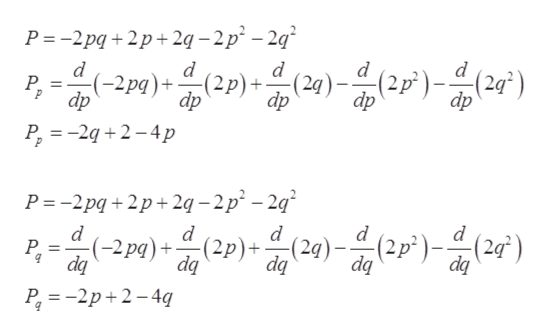# Common blood types are determined genetically by three alleles A, B, and O. (An allele is any of a group of possible mutational forms of a gene.) A person whose blood type is AA, BB, or OO is homozygous. A person whose blood type is AB, AO, or BO is heterozygous. The Hardy-Weinberg Law states that the proportion P of heterozygous individuals in any given population is P(p, q, r) = 2pq + 2pr + 2qr where p represents the percent of allele A in the population, q represents the percent of allele B in the population, and r represents the percent of allele O in the population. Use the fact that p + q + r = 1to show that the maximum proportion of heterozygous individuals in any population is 23.The maximum possible proportion of heterozygous individuals occurs when (p, q, r) = .

Question
33 views

Common blood types are determined genetically by three alleles A, B, and O. (An allele is any of a group of possible mutational forms of a gene.) A person whose blood type is AA, BB, or OO is homozygous. A person whose blood type is AB, AO, or BO is heterozygous. The Hardy-Weinberg Law states that the proportion P of heterozygous individuals in any given population is
P(p, q, r) = 2pq + 2pr + 2qr
where p represents the percent of allele A in the population, q represents the percent of allele B in the population, and r represents the percent of allele O in the population. Use the fact that
p + q + r = 1
to show that the maximum proportion of heterozygous individuals in any population is
2
3
.

The maximum possible proportion of heterozygous individuals occurs when
(p, q, r) =

.

check_circle

Step 1

Given:

Step 2

Solve p + q + r = 1 and put the value of this in above equation:help_outlineImage Transcriptionclosep+q+r =1 r 1-p-q put this value in above equation: P(p.q.r) 2pq+ 2gr +2 pr P 2pq +2q (1-p-9)+2p(1-p-) P 2pq+2-2pq-2q +2p-2p-2pq P =-2pq 2p+ 2q -2p - 2q fullscreen
Step 3

Differentiate with respect to &ls...help_outlineImage TranscriptioncloseP =-2pq+2p+2q -2p -2q pe)(2p) (2)-(2p)(2) dp d d (2р) +. dp d (-2pg)+ P = dp dp P2 224p P =-2pq 2p 2q -2p - 2 d d (24)-(2p)(2) d Р. da 2p)(2p)(29)-2)(2) dq dq dq dq P2-2p2-4q fullscreen

### Want to see the full answer?

See Solution

#### Want to see this answer and more?

Solutions are written by subject experts who are available 24/7. Questions are typically answered within 1 hour.*

See Solution
*Response times may vary by subject and question.
Tagged in

### Calculus